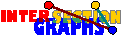# Clique Graphs

We have seen that intersection graphs of Helly-hypergraphs behave well. What about intersection graphs of conformal hypergraphs (i.e. underlying graphs of Helly-hypergraphs)? Note that we may concentrate on simple conformal hypergraphs where no hyperedge contains another, since otherwise we could modify the hypergraph slightly---by adding a new vertex for every hyperedge, contained only in that hyperedge. These hypergraphs are just the clique hypergraphs of graphs. The clique graph C(G) of a graph G is the intersection graph of the family of all cliques of G. Thus the intersection graphs of conformal hypergraphs are just the clique graphs.

Clique graphs seem to be very difficult to deal with. Other than in the Helly-case, not every graph is the intersection graph of some conformal hypergraph (i.e. clique graph) Clique graphs have an obvious dualization, see [RS71] but no good characterization. In other words, clique graphs are very interesting.

## Clique graphs of intersection classes

Although (or maybe since) intersection graphs carry less information than intersection multigraphs, clique graphs of chordal graphs have a nice characterization:[SB94]: A graph G is the clique graph of a chordal graph if and only if G has some spanning tree T such that for every xyE(G), all vertices z on the unique x-y path in T are also adjacent to x and y.

Actually these graphs can be recognized by some O(n2m)-time greedy algorithm [SB94]

Surely, clique graphs of graphs of a Scheinerman class G(P), where P has the Helly property, ave some nice feature: Let G be the intersection graph of certain P-sets Sx. Then the vertices of C(G) correspond to the cliques of G, which themselves correspond to certain points of the union of all Sx. Two such vertices of C(G) are adjacent whenever the corresponding points are covered by some common Sx.

Let us give the criterion for clique graphs of intersection graphs of 2-dimensional boxes. The question is, whether the vertices of the graph H in question can be placed on the plane in such a way that for every two adjacent vertices x and y, the box generated by them covers only vertices of H adjacent to both x and y. Thus we place the vertices of H in such a way that the box generated by the placed vertices of every clique of H contains no further placed vertices of H. This is essentially the first condition of the criterion in Subsection 4.4.Can clique graphs of intersection graphs of 2-dimensional boxes be recognized in polynomial time?

Escalante's characterization [E73] of second iterated clique graphs of so-called clique-Helly graphs also follow by the above observations.

Maybe you want to visit other classes of intersection graphs that are no Scheinerman classes, as intersection graphs of self-dual hypergraphs or competition graphs? Back to the start page for intersection graphs.

Erich Prisner